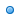Learning 21 Times TablesRelated Math Calculators

Online math number calculation, formulas , Online Algebra calculation, formulas , Matrix calculation, formulas , Digital calculation, Statistical calculation21 Times Tables

An online mathematical 21 times table

21 Addition 21 Subtraction 21 Multiplication 21 Division
1 + 21 = 22 22 - 21 = 1 1 x 21 = 21 21 ÷ 21 = 1
2 + 21 = 23 23 - 21 = 2 2 x 21 = 42 42 ÷ 21 = 2
3 + 21 = 24 24 - 21 = 3 3 x 21 = 63 63 ÷ 21 = 3
4 + 21 = 25 25 - 21 = 4 4 x 21 = 84 84 ÷ 21 = 4
5 + 21 = 26 26 - 21 = 5 5 x 21 = 105 105 ÷ 21 = 5
6 + 21 = 27 27 - 21 = 6 6 x 21 = 126 126 ÷ 21 = 6
7 + 21 = 28 28 - 21 = 7 7 x 21 = 147 147 ÷ 21 = 7
8 + 21 = 29 29 - 21 = 8 8 x 21 = 168 168 ÷ 21 = 8
9 + 21 = 30 30 - 21 = 9 9 x 21 = 189 189 ÷ 21 = 9
10 + 21 = 31 31 - 21 = 10 10 x 21 = 210 210 ÷ 21 = 10
11 + 21 = 32 32 - 21 = 11 11 x 21 = 231 231 ÷ 21 = 11
12 + 21 = 33 33 - 21 = 12 12 x 21 = 252 252 ÷ 21 = 12
13 + 21 = 34 34 - 21 = 13 13 x 21 = 273 273 ÷ 21 = 13
14 + 21 = 35 35 - 21 = 14 14 x 21 = 294 294 ÷ 21 = 14
15 + 21 = 36 36 - 21 = 15 15 x 21 = 315 315 ÷ 21 = 15
16 + 21 = 37 37 - 21 = 16 16 x 21 = 336 336 ÷ 21 = 16
17 + 21 = 38 38 - 21 = 17 17 x 21 = 357 357 ÷ 21 = 17
18 + 21 = 39 39 - 21 = 18 18 x 21 = 378 378 ÷ 21 = 18
19 + 21 = 40 40 - 21 = 19 19 x 21 = 399 399 ÷ 21 = 19
20 + 21 = 41 41 - 21 = 20 20 x 21 = 420 420 ÷ 21 = 20Best Times Tables Generator Here

Our times table creator provides a fun and engaging way for students to learn their times tables.Unlimited Times Table GeneratorCustomize Times Table GeneratorOne click Times Table Answer GeneratorInteractive Times Table Quiz GeneratorOne Hundred ChartGenerate Times TableRecommended Pages21 Times Tables Chart

my math times tables made easy for school children to use and practice series of math times tables from 1 to 21 using this tool. Students can take printable Division 21 times tables, Multiplication 21 times tables, addition 21 times tables and Subtraction 21 times tables from this page.Please hit on print icon and pdf icon to print and download the math times table from mymathtables.com

Practice Times Table 21-100 Chart

Students will learn their times tables quick and easy from mymathtables.com. Everything on this page is easy to print and save lot of time for teachers and parents to focus on helping their students & Kids.